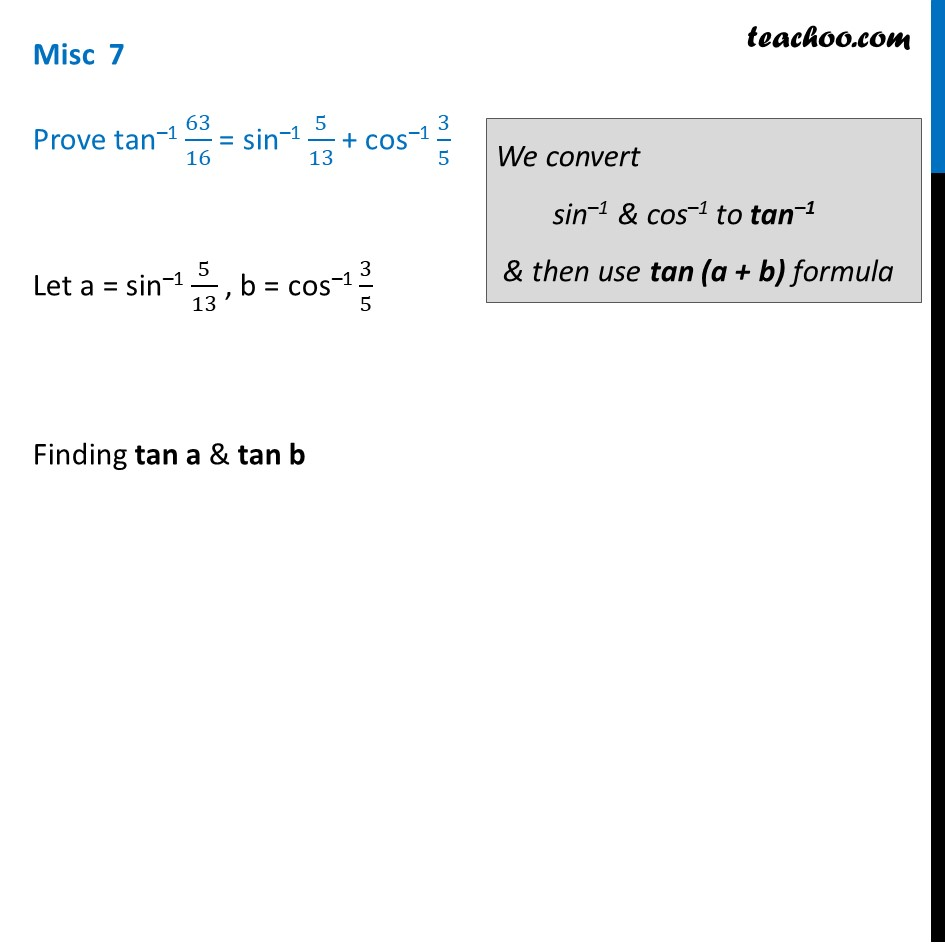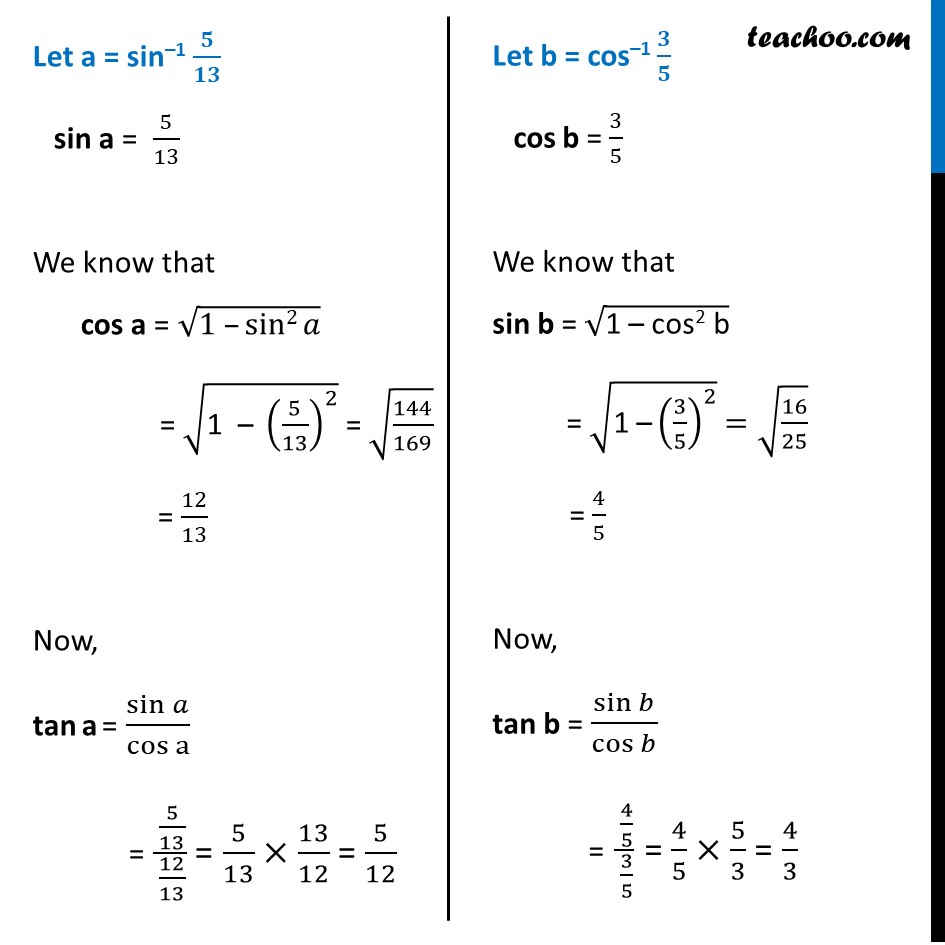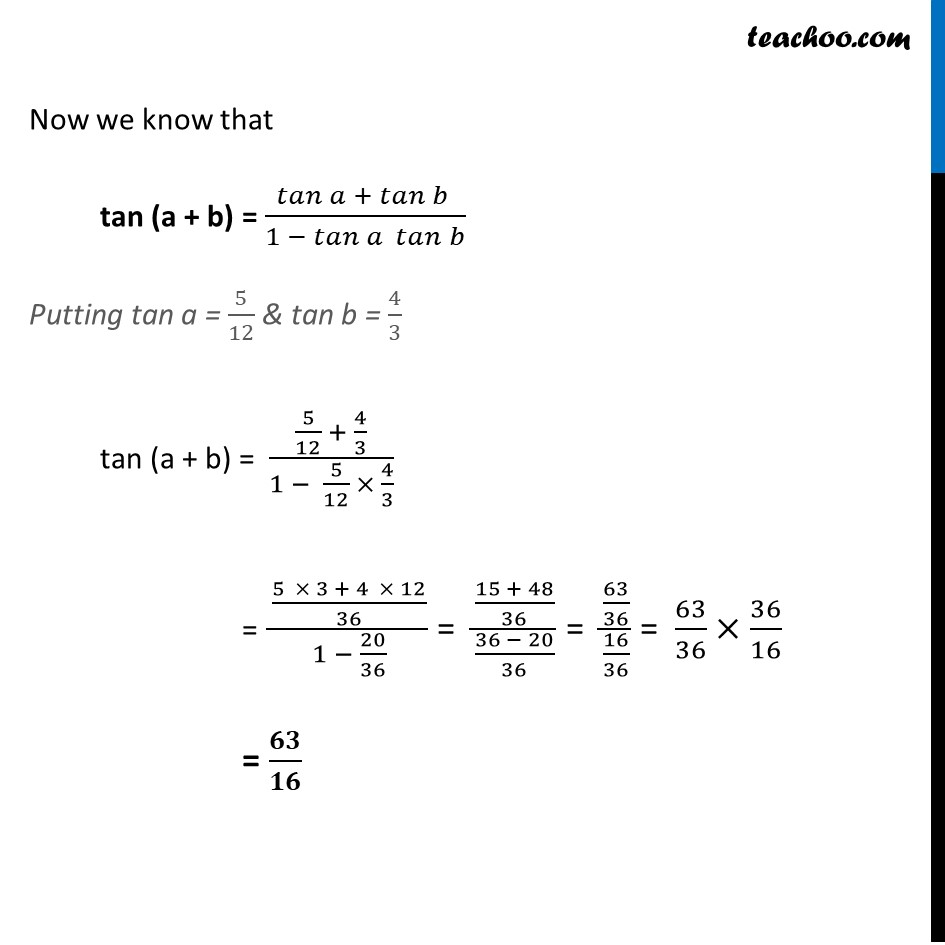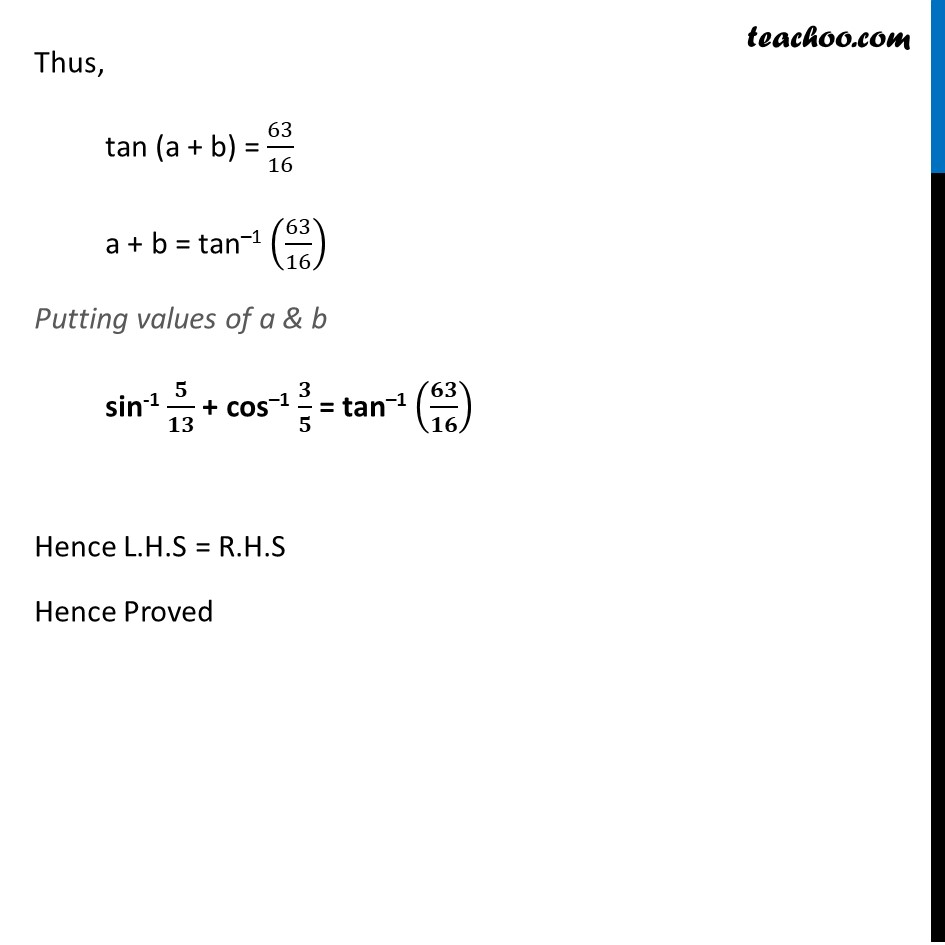Changing of trignometric variables and then applying formula

Chapter 2 Class 12 Inverse Trigonometric Functions
Concept wiseLearn in your speed, with individual attention - Teachoo Maths 1-on-1 Class

### Transcript

Misc 7 Prove tan–1 63/16 = sin–1 5/13 + cos–1 3/5 Let a = sin–1 5/13 , b = cos–1 3/5 Finding tan a & tan b We convert sin–1 & cos–1 to tan–1 & then use tan (a + b) formula Let a = sin–1 𝟓/𝟏𝟑 sin a = 5/13 We know that cos a = √(1 –sin2 𝑎) = √("1 – " (5/13)^2 ) = √(144/169) = 12/13 Now, tan a = (sin 𝑎)/(cos a) = (5/13)/(12/13) = 5/13×13/12 = 5/12 Let b = cos–1 𝟑/𝟓 cos b = 3/5 We know that sin b = √("1 – cos2 b" ) = √("1 –" (3/5)^2 ) =√(16/25) = 4/5 Now, tan b = sin⁡𝑏/cos⁡𝑏 = (4/5)/(3/5) = 4/5×5/3 = 4/3 Now we know that tan (a + b) = 𝑡𝑎𝑛⁡〖𝑎 +〖 𝑡𝑎𝑛〗⁡〖𝑏 〗 〗/(1 − 𝑡𝑎𝑛⁡〖𝑎 𝑡𝑎𝑛⁡𝑏 〗 ) Putting tan a = 5/12 & tan b = 4/3 tan (a + b) = (5/12 + 4/3)/(1 − 5/12 × 4/3) = ((5 × 3 + 4 × 12)/36)/(1 − 20/36) = ((15 + 48)/36)/((36 − 20)/36) = (63/36)/(16/36) = 63/36×36/16 = 𝟔𝟑/𝟏𝟔 Thus, tan (a + b) = 63/16 a + b = tan–1 (63/16) Putting values of a & b sin-1 𝟓/𝟏𝟑 + cos–1 𝟑/𝟓 = tan–1 (𝟔𝟑/𝟏𝟔) Hence L.H.S = R.H.S Hence Proved